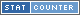TOP  | MISCONS  | GOOD STUFF  | NEW STUFF  | SEARCH
UP to SCIENCE HOBBYIST | UP to LIFTING FORCE MISCONCEPTIONS

# EXPLANATIONS OF AIRPLANE FLIGHT

7/2000 William Beaty
OK, if the "pathlength" explanation is wrong, how can we CORRECTLY explain how an airplane remains aloft? Answer: use one or both of the following explanations. Both are correct, but notice that the "Bernoulli" explanation is a bit more complicated than the "Newton" explanation.

Also note that both explanations require that the wing deflects the air downwards. Obviously the "Newton" explanation depends on this. What most people don't realize is that the "Bernoulli" explanation has the same requirement! In order to create the "circulation" which causes the pressure difference, the air must flow diagonally downwards off of the trailing edge of the airfoil. This is called the "Kutta condition" in the Bernoulli-based mathematics of flight. The downwards flow can be created by tilting an uncambered airfoil. It can also be created by using an un-tilted, cambered airfoil. In other words, CAMBERED AIRFOILS BEHAVE AS IF THEY ARE TILTED. Cambered airfoils deflected air downwards, just as tilted airfoils do.

NEWTONIAN EXPLANATION:

1. Gravity pulls downwards on an airplane.
2. In order to remain aloft, the airplane pushes downwards on the air.
3. The wings have camber and/or are tilted relative to the flowing air.
4. Air moves diagonally downwards off the wing's trailing edge.
5. The wing pushes air downwards, and the airplane is pushed upwards.
6. Gravity cancels out the airplane's upward movement, so the airplane flys horizontally.
7. As the airplane flys, it leaves a trail of downwards-moving air.

BERNOULLI EXPLANATION:

1. The airplane flies forwards.
2. The wing has camber and/or is tilted relative to the flowing air.
3. Air has inertia, so the air approaching the wing flows differently than the air which leaves the trailing edge of the wing.
4. Air moves diagonally downwards off the wing's trailing edge.
5. The downwards motion causes the air to "circulate" around the entire wing. It's like a tornado, with the wing at its center.
6. Above the wing, this "circulation" adds to the velocity of the oncoming air, so that air above the wing flows faster.
7. Below the wing, the "circulation" subtracts from the velocity of the oncoming air, so that air below the wing flows slower by an equal amount.
8. BERNOULLI'S EQUATION describes the faster air as having lower pressure.
9. The difference in the air speed above and below the wing causes a difference in pressure.
10. The pressure-difference lifts the airplane.

MORE NEWTON

While remaining aloft, a 1000KG airplane gains downwards momentum because of gravity. F=MA=(MV)/SEC, so the airplane gains 9800 KG-METERS of downwards momentum for every second it remains suspended. It must apply a downwards force to the air in order to experience an upward force, and as a result the airplane injects its downwards momentum into the air. The airplane doesn't fall, instead the air being intercepted by the wings is thrown downwards, and it "falls" in place of the airplane. The airplane rids itself of 9800KG-M of momentum per second. Knowing this, we can calculate the net downwards velocity of the air in the wake behind the plane. If the wings intercept 100KG of air per second, then that air must move downwards at 98 meters per second in order to carry away the momentum and keep the plane flying level. If the airplane flys faster and intercepts 1000KG of air per second, then that air need only move downwards at 9.8 M/S in order to keep the plane flying.

EVEN MORE NEWTON

A 1000KG airplane which deflects air downwards at a velocity of 10 meters per second must deflect 980KG of air per second and expend 49 kilowatts in order to fly. F = MA = MV/SEC, and E = 1/2MV^2

An airplane of similar weight, if it deflects air downwards at a velocity of only 1 meter per second, must deflect 9800KG of air per second in order to fly. That's ten times more air deflected (the wings would be much longer than in the case of the first airplane.) But this one only expends 4.9 kilowatts to deflect the air.

The moral: use longer wings and wider jet engine intakes in order to gently accelerate the air. The airplane performs less work to swirl the air, and this saves gasoline. The longer the wings, the less work performed to fight gravity. Or, if you fly faster, you can intercept more air per second and accelerate it to a lower speed, and therefor high-speed flight uses far less energy. This effect is usually called "induced drag." The "bernoulli-based" theory of flight doesn't make these facts very obvious.

## OTHER GOOD WEBSITES

```

http://amasci.com/wing/wingexp.html
Created and maintained by Bill
Beaty.  Mail me at:.

var sc_project=10408414;
var sc_invisible=0;
var sc_security="00267183";
var scJsHost = (("https:" == document.location.protocol) ?
"https://secure." : "http://www.");
document.write("<sc"+"ript type='text/javascript' src='" + scJsHost + "statcounter.com/counter/counter.js'></"+"script>");View My Stats

```